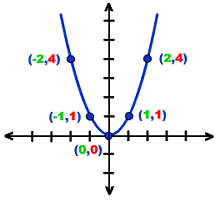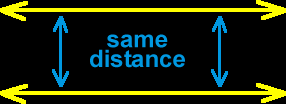# Online Math Dictionary: P

Easy to understand math definitions for K-Algebra mathematics
Just scroll down or click on the word you want and I'll scroll down for you!

 parabola parallel parallelogram Pascal's triangle pentagon pentagram percent perimeter permutations perpendicular pi piecewise function point polygon polyhedron polynomial positive numbers prime prism probability product proof proper fraction proportion pyramid Pythagorean Identities Pythagorean Theorem Pythagorean triples

 ParabolaThe formula for the standard parabola isFor more info and examples, check out myParabola lesson. For graphing parabolas, check out my Graphing Parabolas lessons (first in a series).ParallelTwo lines (lying in the same plane) are parallel if they never intersect...  This means that the two lines are always the same distance apart.ParallelogramA parallelogram is a quadrilateral (a four sidedpolygon) where both pairs of opposite sides are parallel. For more info, check out Properties of Parallelograms.